Lesson 60: Plane Mirrors

“Plane” in this case refers to “flat.” Plane mirrors are just basic, regular mirrors.

• If the mirrors were not flat we would have to use more complicated rules (more on those in Lesson 61).

Doing problems involving plane mirrors is actually pretty easy since we only have to remember a few things:

1. The Law of Reflection. Any light beam that hits the mirror will bounce off at exactly the same angle. We assume the mirror is perfect flat in these situations. We will have to make sure that the light rays reflected off the mirror hit the observers eye.
2. The image will be the same size as the original object
3. The image will appear as far behind the mirror as the object is in front of the mirror.

The only challenge (and it’s not a very big one) is figuring out how your eye/brain interprets the information and where the reflected object appears to be.

• Let’s look at a simple example to illustrate how I would draw it.

Example 1: Sketch a ray diagram that shows how light will travel from the object to the eye by reflecting from the mirror in the given diagram. Show the position of the image.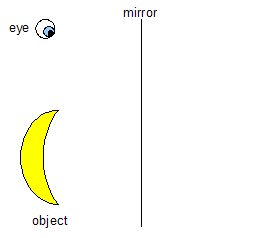Figure 1

First, measure how far the object is in front of the mirror. Draw a quick sketch of the image behind the mirror at the same distance (just make sure it’s flipped around, and should be drawn with a dashed line).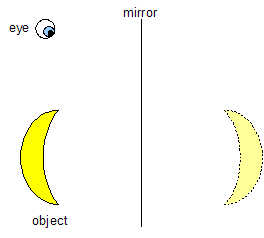Figure 2

Draw a light ray (a line) from the observer’s eye to an important part of the image (like the top). The light ray should be dashed when it is behind the mirror to show that the light ray isn’t really there.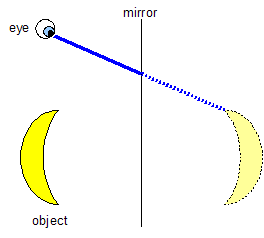Figure 3

At the point where that light ray hit the mirror, bounce it back at the same angle (law of reflection) so that it hits the same spot on the original object.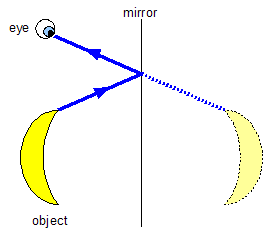Figure 4

Now try to draw another separate light ray that shows the path the light takes to get from the bottom of the object to the eye. You should get something that looks like this: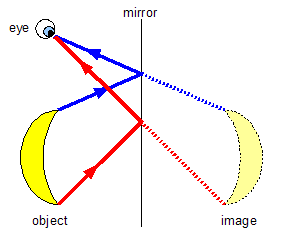Figure 5

This gives you an idea of how the light rays are traveling from the object to you eye, and also why the image appears to be behind the mirror.

• Because the image isn’t really a "thing" behind the mirror (meaning that there are no true light rays that are back there), we refer to it as a “virtual image”.
• That's why we drew anything behind the mirror as a dotted line. It shows that there are no real rays of light back there.
• You can always do a test in your head to determine if you are dealing with a virtual image. Ask yourself “If I put a piece of paper where I think the image is (in the example above that would be behind the mirror), will I see the image on the paper like a film on a movie screen?” If the answer is “no”, you have a virtual image.
• Sometimes the answer can be “yes” when dealing with curved mirrors, the topic of Lesson 61.